### Home > MC2 > Chapter 5 > Lesson 5.1.2 > Problem5-24

5-24.
1. The scale drawing below shows the first floor of a house. The actual dimensions of the garage are 20 feet by 25 feet. All angles are right angles. Homework Help ✎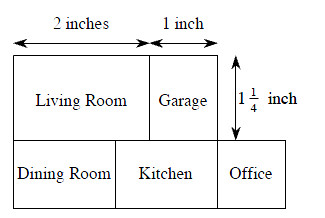1. How many feet does each inch represent?

2. What is the length and width of the living room in inches?

3. What is the length and width of the living room in feet?

4. What is the area of the living room (in square feet)?

5. What is the perimeter of the garage (in feet)?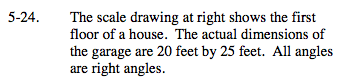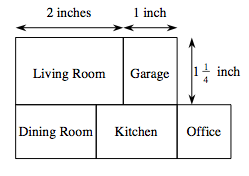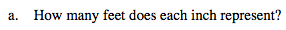Consider the actual dimensions of the garage as a similar shape. Find the ratio of the scale factor between the actual garage and the scale drawing at right.

20 feet = 1 inch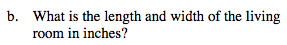Refer to the drawing below. The living room is in the upper left corner of the house. Its width is the same as the garage.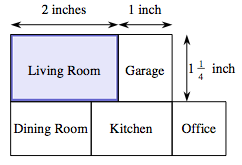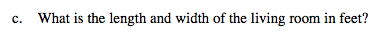Use the scale drawing dimensions from part (b) and the scale factor for feet from part (a).

40 feet by 25 feet.
Make sure you know why this is the answer.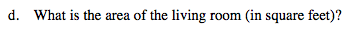Use your answer from part (c) to determine the actual area of the living room (in square feet).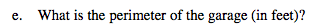Refer to parts (a) through (c) for help.